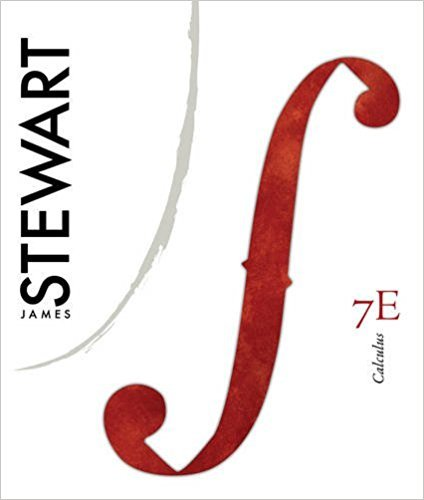×
Get Full Access to Calculus, - 7 Edition - Chapter 14 - Problem 11
Get Full Access to Calculus, - 7 Edition - Chapter 14 - Problem 11

×

# A metal plate is situated in the -plane and occupies theISBN: 9780538497817 125

## Solution for problem 11 Chapter 14

Calculus, | 7th Edition

• Textbook Solutions
• 2901 Step-by-step solutions solved by professors and subject experts
• Get 24/7 help from StudySoup virtual teaching assistantsCalculus, | 7th Edition

4 5 1 420 Reviews
17
4
Problem 11

A metal plate is situated in the -plane and occupies the rectangle , , where and are measured in meters. The temperature at the point in the plate is , where is measured in degrees Celsius. Temperatures at equally spaced points were measured and recorded in thetable.(a) Estimate the values of the partial derivativesand . What are the units?(b) Estimate the value of , where .Interpret your result.(c) Estimate the value of .

Step-by-Step Solution:
Step 1 of 3

Step 2 of 3

Step 3 of 3

##### ISBN: 9780538497817

This textbook survival guide was created for the textbook: Calculus,, edition: 7. Since the solution to 11 from 14 chapter was answered, more than 252 students have viewed the full step-by-step answer. This full solution covers the following key subjects: Where, estimate, measured, plate, point. This expansive textbook survival guide covers 17 chapters, and 887 solutions. The full step-by-step solution to problem: 11 from chapter: 14 was answered by , our top Calculus solution expert on 11/10/17, 05:23PM. The answer to “A metal plate is situated in the -plane and occupies the rectangle , , where and are measured in meters. The temperature at the point in the plate is , where is measured in degrees Celsius. Temperatures at equally spaced points were measured and recorded in thetable.(a) Estimate the values of the partial derivativesand . What are the units?(b) Estimate the value of , where .Interpret your result.(c) Estimate the value of .” is broken down into a number of easy to follow steps, and 73 words. Calculus, was written by and is associated to the ISBN: 9780538497817.

Unlock Textbook Solution